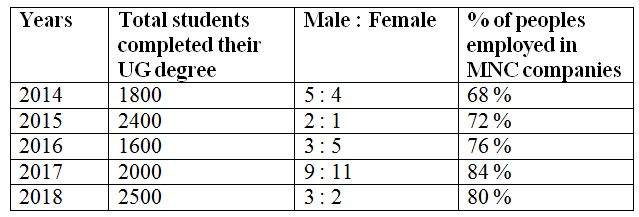# gkrecall

We brings you daily Current affairs, daily Current Affairs Quiz, weekly Current Affairs, weekly Current Affairs Quiz to enhance your preparation for upcoming exams. We also provide articles related to quant, reasoning, English, ssc etc .

## QUANT PRACTICE SET 3 FOR RRB PO & CLERK PRELIM 2020

RRB Clerk and RRB PO Prelims exam are going to be held in the upcoming months. We have already provided you with the PDFs of many topics of Quantitative Aptitude like Simplification/Approximation, Number Series,datainterpretation.important Arithmetic questions to prepare for RRB Clerk and PO Pre exam. Practicing these questions will help you to know about the level of the questions. To increase your speed and accuracy, enhance your calculations.

Web

### START QUIZ

Directions (Q. 1 – 5): In the following questions, two equations I and II are given. You have to solve both the equations and give Answer as,
a) If x > y
b) If x ≥ y
c) If x < y
d) If x ≤ y
e) If x = y or the relation cannot be established
1)
I) 2x2 – 16x + 32 = 0
II) 3y2 – 15y + 18 = 0
2)
I) x2 – 4x – 77 = 0
II) y2 – 27y + 92 = 0
3)
I) 4x + 5y + 26 = 0
II) 3x – 4y + 4 = 0
4)
I) 3x2 + 14x + 15 = 0
II) 3y2 – 13y + 14 = 0
5)
I) 4x2 – 6x – 18 = 0
II) 5y2 + 6y – 27 = 0
Directions (Q. 6 – 10): Study the following information carefully and answer the given questions?
The following table shows the total number of students completed their UG degree in different years in a certain college and the ratio of male and female. The percentage of peoples employed in MNC companies and the remaining peoples are doing own business.6) Find the ratio between the total number of students employed in MNC companies in the year 2014 and the total number of peoples who are doing business in 2018?
a) 155 : 79
b) 217 : 113
c) 346 : 251
d) 52 : 15
e) None of these
7) Find the average number of students who completed their UG degree in all the given years together?
a) 1820
b) 1640
c) 2280
d) 2060
e) None of these
8) Find the difference between the total number of males who completed their degree in the year 2015 to that of total number of females who completed their degree in the year 2017?
a) 500
b) 750
c) 600
d) 900
e) None of these
9) The total number of peoples employed in MNC companies in the year 2015 is approximately what percentage of total number of peoples employed in MNC companies in the year 2017?
a) 126 %
b) 88 %
c) 103 %
d) 147 %
e) 165 %
10) The total number of peoples who completed their UG degree in 2016 and doing business is what percentage more/less than the total number of female students who completed their UG degree in the year 2014?
a) 36 % more
b) 52 % less
c) 36 % less
d) 52 % more
e) 64 % more

SOLUTIONS:
Directions (1-5):
I) 2x2 – 16x + 32 = 0
2x2 – 8x – 8x + 32 = 0
2x (x – 4) – 8 (x – 4) = 0
(2x – 8) (x – 4) = 0
x = 8/2, 4 = 4, 4
II) 3y2 – 15y + 18 = 0
3y2 – 9y – 6y + 18 = 0
3y (y – 3) – 6 (y – 3) = 0
(3y – 6) (y – 3) = 0
y = 6/3, 3 = 2, 3
x > y
I) x2 – 4x – 77 = 0
(x – 11) (x + 7) = 0
x = 11, -7
II) y2 – 27y + 92 = 0
(y – 23) (y – 4) = 0
y = 23, 4
Can’t be determined
4x + 5y = – 26 –> (1)
3x – 4y = – 4 –> (2)
By solving the equation (1) and (2), we get,
x = -4, y = -2
x < y
I) 3x2 + 14x + 15 = 0
3x2 + 9x + 5x + 15 = 0
3x (x + 3) + 5 (x + 3) = 0
(3x + 5) (x + 3) = 0
x = -5/3, -3
II) 3y2 – 13y + 14 = 0
3y2 – 6y – 7y + 14 = 0
3y (y – 2) – 7 (y – 2) = 0
(3y – 7) (y – 2) = 0
y = 7/3, 2
x < y
I) 4x2 – 6x – 18 = 0
4x2 – 12x + 6x – 18 = 0
4x(x – 3) + 6(x – 3) = 0
(4x + 6) (x – 3) = 0
x = -6/4, 3 = -3/2, 3 = -1.5, 3
II) 5y2 + 6y – 27 = 0
5y2 + 15y – 9y – 27 = 0
5y(y + 3) – 9(y + 3) = 0
(5y – 9) (y + 3) = 0
y = 9/5, -3 = 1.8, -3
Can’t be determined
Direction (6-10) :
The total number of students employed in MNC companies in the year 2014
= > 1800*(68/100)
The total number of students who completed their UG degree in 2018 and doing business
= > 2500*(20/100)
Required ratio = [1800*(68/100)] : [2500*(20/100)]
= > 306 : 125
The average number of students who completed their UG degree in all the given years together
= > (1800 + 2400 + 1600 + 2000 + 2500) / 5
= > (10300/5) = 2060
The total number of males who completed their degree in the year 2015
= > 2400*(2/3) = 1600
The total number of females who completed their degree in the year 2017
= > 2000*(11/20) = 1100
Required difference = 1600 – 1100 = 500